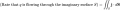# The Continuity Equation

#### rahulpsharma

Joined Sep 5, 2010
38
Continuity equation - Wikipedia

I have a question in the 'General Equation, Definition of Flux and Integral Form' paras...!!

The description till the following is understood:But then suddenly in the next section (Integral Form), it is stated:
"Rate of q PLUS the surface integral of j.ds = sigma"

My question is:
Shouldnt it follow from the earlier description that:

"Rate of q MINUS the surface integral of j.ds = sigma"

I mean didnt we just equate dq/dt to surface integral of flux...??

If x = y, then further algebraic manipulation would lead to x - y = 0

So why in the following line the two terms are added...!! Whatever is present inside the surface integral is EQUAL to dq/dt...!!

Regards